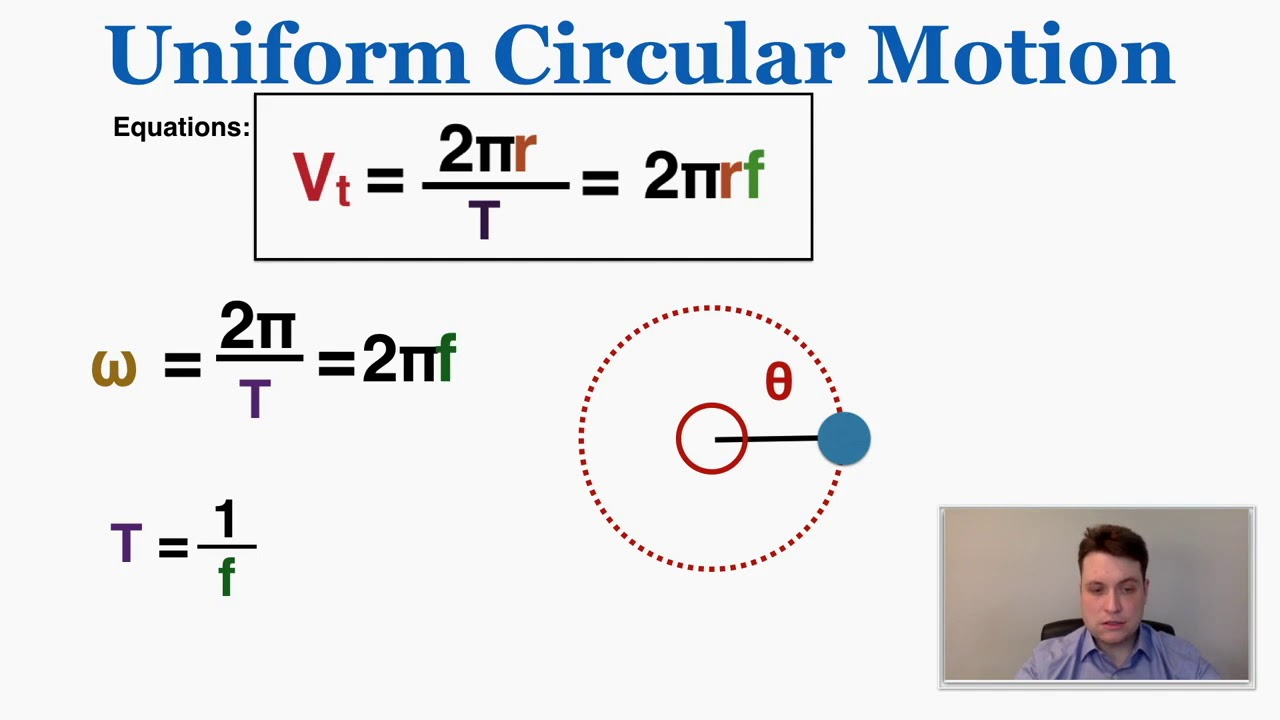# How can I solve circular motion physics problems?

Physics is a very important subject as it explores how space and matters move and behave. Understanding the basics of physics will help a student further down the road in their academic venture as the more advanced concepts of physics that are taught in colleges and higher institutions all have their foundation on the basics of physics like the law of motion, law of thermodynamics, and much more. Physics is mostly introduced in the later part of a student’s secondary education from the eighth standard and the basics are studied in depth from the 11th standard onwards. Moreover, like math, physics is a concept-based subject. Students cannot simply study physics like that of civics, history, or language. They need to understand the concepts and theories thoroughly in order to be able to able to solve the physics problem that will enable the students to achieve high marks in their exams. Also, understanding the theories and concepts deeply will really help in the later stages of students’ educational path in their undergraduate, post-graduate, and so on. So, the students need to make sure they spend time studying this subject in order to do well in their studies.

The syllabus of physics is vast but if the students learn the concepts well, they will face difficulty in studying the subject. One important concept in physics for the 12th board exam is “Circular Motion”. It is not a difficult concept. Students just need to focus and clearly understand the concept and working principle of circular motion. Let us dive deeper into this topic:

### Circular Motion

Circular motion is defined as the movement of an item along a circular route while spinning. Circular motion can be uniform or non-uniform in nature. The angular rate of rotation and speed is constant in a uniform circular motion, while the rate of rotation changes in non-uniform motion.

Listed below are some examples of circular motion:

• Revolution of the satellite around the earth.
• The motion of the car wheels while moving.
• Gears are located in gas turbines.
• The motion of the blades of a windmill.
• Rotation of a ceiling fan.

When a particle goes around the perimeter of a circular route, it is said to be in a circular motion. Unlike linear motion, the direction of motion that changes continually is a fundamental feature of circular motion. As a result, angular variables may be used to define circular motion.

Listed below are some of the different variables associated with circular motion:

• Angular Displacement: It is the angle that a spinning particle turns in one unit of time.
• Angular Velocity: It’s the rate at which a particle’s angular displacement changes in a circular motion.
• Angular Acceleration: It is defined as the rate at which the rotating particle’s angular velocity changes. The motion that is circular can either be non-uniform or uniform based on the type of acceleration of the particle. When a particle moves along a circular route at a constant speed, the motion is called uniform circular motion. The velocity vector changes direction at each point on the circle at the time of a circular motion. As a result, the radial component of acceleration is never zero. In the event of non-uniform circular motion, the tangential component might be positive or negative, and in the case of uniform circular motion, it can be zero.